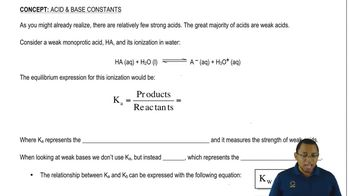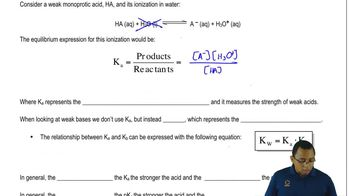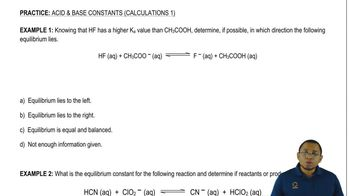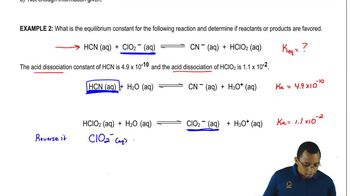Start typing, then use the up and down arrows to select an option from the list.Jules Bruno
200views
2
2
So here we have to determine which of the following solutions will have the lowest pH. So lowest pH here really means strongest acid. If we take a look at all of these compounds were gonna realize that all of them having them hydrogen oxygen and a non metal So they're all oxy acids. So what we have to do first is figure out how many oxygen's we have remaining in all of them. The one with the most oxygen's remaining would be the strongest oxy acid. So here we have to oxygen's and one hydrogen. So we have one oxygen left when we subtract them here, we have a child. Four. So we have four oxygen's in one hydrogen. Subtract them. We have three oxygen's left, three oxygen's and six hydrogen is we have no oxygen is left here. Three oxygen's to hydrogen. So one oxygen left and then four oxygen's and one hydrogen. Three oxygen is left. So we know that we're gonna be looking at We're gonna be looking at B and E because they have the most options remaining. Now, remember the final one here It has a negative charge, so it has ah, hydrogen and it has a negative charge, which means it acts as an AMFA Terek species. Remember, it can act as an acid or base because of this anfo Terek species where you will not consider being strong acids. So here it's week, so it's eliminated here. H I 04 is a strong acid that's given. So it is our answer here. Okay, so since it's the strongest acid, it will produce the lowest pH out of the choices given.01:2003:5101:3407:0901:53